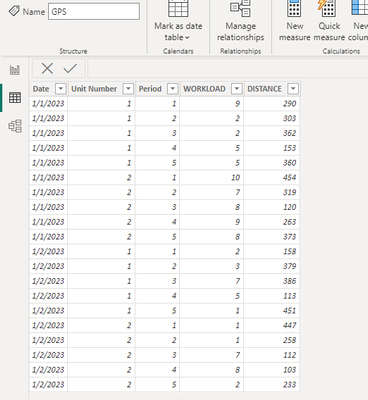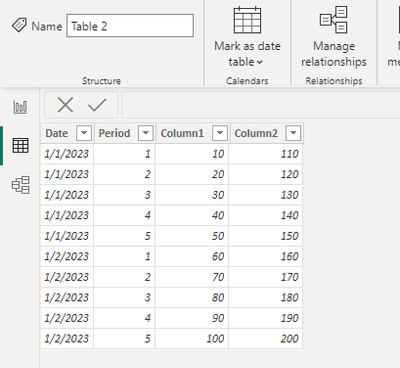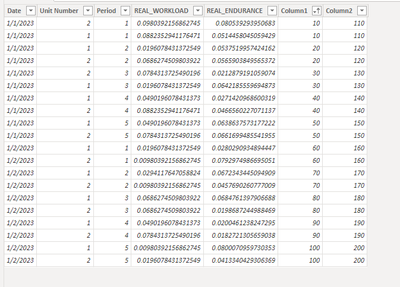cancel
Showing results for
Did you mean:Frequent Visitor

## How to merge columns from different tables in a new table caculation not displayed in Power Query

Hi,

I created a new table (table 3) with some measures from another table (table 1) and now i need to add 3 collumns from a third table (table 2) through mulple relationships (i.e., date, and moment of the day).

I know how to merge columns from different tables with Power Query but the new table created (table 3) is not displayed in Power Query (maybe because it is not imported externally).

What are the best solutions:

1) How to add columns from 2 different tables?

This is the code of the created new table (table 3):
GPS, GPS[Date], GPS[Unit Number], GPS[Period]),
"REAL_ENDURANCE", GPS[%_TOTAL DISTANCE],
"REAL_SHORT", DIVIDE([%_ACC&DEC>2]+[%_ACC&DEC>3],2),
"REAL_LONG", DIVIDE([%_HSD-Z5]+[%_SD-Z6],2))

2) How to dynamically merge columns from 2 different tables with at least 2 relationships without Power Query?

Thanks

SQ

1 ACCEPTED SOLUTIONCommunity Support

You can achieve this by using ADDCOLUMNS and FILTER()

Here is may example:``````RealxExpected =
SUMMARIZE ( GPS, GPS[Date], GPS[Unit Number], GPS[Period] ),
"REAL_ENDURANCE", GPS[%_TOTAL DISTANCE],
"Column1",CALCULATE(MAX('Table 2'[Column1]),FILTER('Table 2','Table 2'[Date]=EARLIER(GPS[Date])&&[Period]=EARLIER(GPS[Period]))),
"Column2",CALCULATE(MAX('Table 2'[Column2]),FILTER('Table 2','Table 2'[Date]=EARLIER(GPS[Date])&&[Period]=EARLIER(GPS[Period])))
)
``````

Final output:2 REPLIES 2Frequent Visitor

It works! Thanks!

SQCommunity Support

You can achieve this by using ADDCOLUMNS and FILTER()

Here is may example:``````RealxExpected =
SUMMARIZE ( GPS, GPS[Date], GPS[Unit Number], GPS[Period] ),
"REAL_ENDURANCE", GPS[%_TOTAL DISTANCE],
"Column1",CALCULATE(MAX('Table 2'[Column1]),FILTER('Table 2','Table 2'[Date]=EARLIER(GPS[Date])&&[Period]=EARLIER(GPS[Period]))),
"Column2",CALCULATE(MAX('Table 2'[Column2]),FILTER('Table 2','Table 2'[Date]=EARLIER(GPS[Date])&&[Period]=EARLIER(GPS[Period])))
)
``````

Final output: